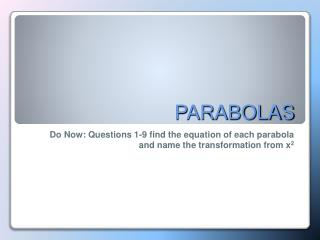DownloadDownload PresentationPARABOLAS

# PARABOLAS

Télécharger la présentation## PARABOLAS

- - - - - - - - - - - - - - - - - - - - - - - - - - - E N D - - - - - - - - - - - - - - - - - - - - - - - - - - -
##### Presentation Transcript

1. PARABOLAS Do Now: Questions 1-9 find the equation of each parabola and name the transformation from x2

2. A parabola is the set of all points equidistant from a fixed line, called the Directrix, and a fixed point not on the line, called the focus. • Equation of a parabola • y = a(x - h)2 + k • The vertex is at point (h, k) • The axis of symmetry is the line x = h • The graph of y = ax2 opens upward if a > 0 and downward if a < 0. • The larger the value of | a | is, the narrower the graph of the parabola is. Things to know about parabolas

3. An object is launched at 19.6 meters per second (m/s) from a 58.8-meter tall platform. The equation for the object's height s at time t seconds after launch is s(t) = –4.9t2 + 19.6t + 58.8, where s is in meters. When does the object strike the ground? TIME FOR THE HARD STUFF

4. STUDENT WORK

5. 0 = –4.9t2 + 19.6t + 58.8 0 = t2 – 4t – 12 0 = (t – 6)(t + 2) The object strikes the ground six seconds after launch. ANSWER

6. An object in launched directly upward at 64 feet per second (ft/s) from a platform 80 feet high. What will be the object's maximum height? When will it attain this height? #2 

7. STUDENT WORK

8. Equation: s(t) = –16t2 + 64t + 80 h = –b/2a = –(64)/2(–16) = –64/–32 = 2 k = s(2) = –16(2)2 + 64(2) + 80 = –16(4) + 128 + 80 = 208 – 64 = 144 It takes two seconds to reach the maximum height of 144 feet. ANSWER

9. s(t) represents the projectile's instantaneous height at any time t • vo represents initial velocity • t represents time in seconds after the projectile is released Notes On “Projectile Problems”

10. A ball is thrown directly upward from an initial height of 200 feet with an initial velocity of 96 feet per second. After how many seconds will the ball reach its maximum height? And, what is the maximum height? EXAMPLE

11. After Mrs. Dron got hit in the head with a pitch, Mr. Chester came up to bat. The equation h=-.004d2 +.96d+3 represents the path the ball took after Mr. Chester hit the first pitch. How far off the ground was the ball when it was hit by the bat? Pair Share

12. h=-.004d2 +.96d+3 h=-.004(0) + .96(0) + 3h=  0 + 0 + 3h= 3 feet, which is how far from the ground the ball was when it was hit by the bat Answer

13. Transformation Worksheet Homework